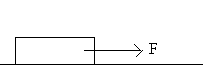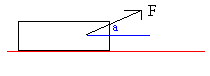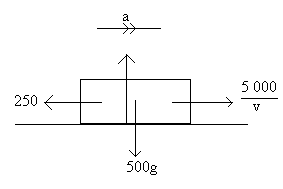## Work, Energy & Power

This section covers Work, Energy and Power using maths.

Work Done

Suppose a force F acts on a body, causing it to move in a particular direction. Then the work done by the force is the component of F in the direction of motion × the distance the body moves as a result. Work done is measured in joules (which has symbol J).So if we have a constant force of magnitude F newtons, which moves a body a distance s (meters) along a flat surface, the work done is F × s joules.Now suppose that this force is at an angle of a to the horizontal. If the body moves a distance of s metres along the ground, then the work done is F cosa × s (since F cosa is the component of the force in the direction of motion).

Work Done Against Gravity

Now suppose that the force we are considering is one which causes a body to be lifted off of the ground. We call the work done by the force the "work done against gravity". This is equal to mgs joules, where s is the vertical distance moved by the body, m is the mass of the body and g is the acceleration due to gravity. [Compare with "gravitational potential energy" below].

Energy

The energy of a body is a measure its ability to do work.

Kinetic Energy

The kinetic energy (K.E.) of a body is the energy a body has as a result of its motion. A body which isn't moving will have zero kinetic energy, therefore.

• K.E. = ½ mv2

where m is the mass and v is the velocity of the body.

Gravitational Potential Energy

Gravitational potential energy (G.P.E.) is the energy a body has because of its height above the ground.

• G.P.E. = mgh

where h is the height of the body above the ground.

There are also other types of potential energy (such as elastic potential energy). Basically, the total potential energy measures the energy of the body due to its position.

Conservation of Energy

If gravity is the only external force which does work on a body, then the total energy of the body will remain the same, a property known as the conservation of energy.

Therefore, providing no work is done:

• Initial (PE + KE) = final (PE + KE)

Connection Between Energy and Work Done

If energy is not conserved, then it is used to do work.

In other words, the work done is equal to the change in energy. For example, the work done against gravity is equal to the change in the potential energy of the body and the work done against all resistive forces is equal to the change in the total energy.

Power

Power is the rate at which work is done (measured in watts (W)), in other words the work done per second.

It turns out that:

• Power = Force × Velocity

For example, if the engine of a car is working at a constant rate of 10kW, the forward force generated is power/velocity = 10 000 / v, where v is the velocity of the car (the 10 was changed to 10 000 so that we are using the standard unit of W rather than kW).

Example

A car of mass 500kg is travelling along a horizontal road. The engine of the car is working at a constant rate of 5kW. The total resistance to motion is constant and is 250N. What is the acceleration of the car when its speed is 5m/s?The equation of motion horizontally (from Newton's Second Law):

5000 - 250 = 500a
v

when v = 5:
500a = 750
a = 1.5

so the acceleration is 1.5ms-2